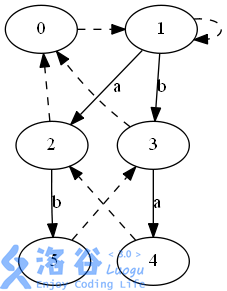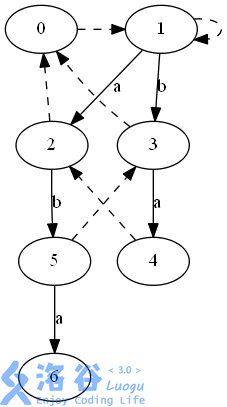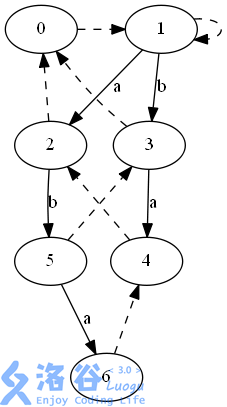回文自动机一一处理回文串问题的有力武器

1.概念

$\quad$a.大概： 同其他自动机一样，回文自动机是个DAG，它用相当少（$O(n)$）的空间复杂度就存储了这个字符串的所有回文串信息。一个回文自动机包含不超过$|S|$个节点，每个节点都表示了这个字符串的一个不重复的回文子串，同时一个节点会有不超过字符集大小的边连向其他节点，以及一条fail边连向这个点的fail...这些都会在下面介绍

$\quad$b.森林： 和别的自动机不太一样，回文自动机是有两棵树的森林：其中一棵是长度为偶数的回文串集合，另一棵是长度为奇数的回文串集合，这两棵树的根节点分别表示长度为0（空串）和-1（无实际含义，便于运算）的回文串;

$\quad$c.边：自动机中每条有向边都有一个字符类型的权值，起点的串左右分别加上这个字符得到的就是终点的串。举个栗子：设一条边权为$c$的边连接的两个点分别是$A,B$$A$表示回文串$aba$，则$B$表示的回文串就是$cabac$ 。特别的，如果$A$是那个长度为$-1$的根，$B$串就是这条边的权值。。。

$\quad$d.点：当你插入一个字符的时候，插入的点代表的就是这个字符匹配的最长回文串，也就是说从根节点往下顺着边走，记着一个str一开始为空，一边走一边不停地往str左右两边添加新的字符，走到一个点，这个点代表的回文串就是str

$\quad$e.$fail$边：每个点都有个fail边，这条边指向这个点所代表的回文串的 最长回文后缀 所在的那个点（最长回文后缀：串中满足回文的最长的后缀，这个串自己不算）如果没有，则指向0（就是那个根节点）。特别的，0的fail节点就是那个长度为-1的点。

2.构造：

$\quad$我是用的一个结构体存的，$len,fail,son,siz$ 分别代表这个串的长，fail节点，连出来的每一条边以及这个回文串的数量，如下

struct node{
int len,fail,son,siz;
};
node prt[maxn];

prt.len=-1;
prt.fail=prt.fail=1;

int getfail(int x){
while(s[n-prt[x].len-1]!=s[n]) x=prt[x].fail;
return x;
}

$cur$已经包含权值为x的出边了，我们就可以简单地将出边终点的权值++，继续去加下一个点了。如果不包含权值x的边，我们就需要新建一个点$now$并让$cur$把边连向他，$now$代表的长度自然是$cur$的长度+2，然后我们只要求出$now$$fail$就完事了。

$fail$的话可以用cur的$fail$来求，就用上面求$cur$的方法，但是不能用$cur$本身（想一想，为什么）

void extend(int x){
int cur=getfail(last);
int now=prt[cur].son[x];
if(!now){
now=++num;
prt[now].len=prt[cur].len+2;
prt[now].fail=prt[getfail(prt[cur].fail)].son[x];
prt[cur].son[x]=now;
}
prt[now].siz++;
last=now;
}

void count(){
for(int i=num;i>=2;i--)
prt[prt[i].fail].siz+=prt[i].siz;
}

4.举个栗子：6 的长度自然是5的长度+2$(a'bab'a)$$siz(6)=1$$siz(4)=1$

$siz(5)=1$

$(siz(4)+=siz(6))=2$

$(siz(3)+=siz(5))=2$

$(siz(2)+=siz(4))=3$

附：闲得自己也写了个造图的代码。。。

void print(int x){
if(cz[x]) return;
cz[x]=1;
for(int i=0;i<=25;i++)
if(sam[x].ch.count(i))
printf("	%d->%d[label=%d];\n",x,sam[x].ch[i],i),
print(sam[x].ch[i]);
}
void Vz(){
printf("digraph zhy{\n	rankdir = LR;\n");
print(0);
printf("}\n");
}

5.例题（Luogu-1659）：

$\quad$这道题的话就是把这些点按照长度从大到小排一遍序，然后前$k$个奇数长的乘起来就是答案啦，注意这题k较大，还要用快速幂，代码：

#include<cstdio>
#include<cstring>
#include<algorithm>
using namespace std;
typedef long long ll;
const int maxn=1e6+100,P=19930726;
struct node{
int len,fail,son,siz;
node(){
len=fail=0;
for(int i=0;i<=25;i++)
son[i]=0;
}
};
node prt[maxn];
int n,last,len,num;
ll ans=1,k;
char s[maxn];
ll poww(ll x,int y){
ll base=1;
while(y){
if(y&1) base*=x,base%=P;
x*=x,x%=P;
y>>=1;
}
return base;
}
bool cmp(node x,node y){
return x.len>y.len;
}
int getfail(int x){
while(s[n-prt[x].len-1]!=s[n]) x=prt[x].fail;
return x;
}
void extend(int x){
int cur=getfail(last);
if(!prt[cur].son[x]){
int now=++num;
prt[now].len=prt[cur].len+2;
prt[now].fail=prt[getfail(prt[cur].fail)].son[x];
prt[cur].son[x]=now;
}
prt[prt[cur].son[x]].siz++;
last=prt[cur].son[x];
}
int main(){
scanf("%d%d",&len,&k);
scanf("%s",s);
last=num=1,prt.len=-1;
prt.fail=prt.fail=1;
for(n=0;n<len;n++) extend(s[n]-'a');
for(int i=num;i>=2;i--)
prt[prt[i].fail].siz+=prt[i].siz,prt[prt[i].fail].siz%=P;
sort(prt+1,prt+num+1,cmp);
int now=1;
while(k){
if(now>num){
printf("-1\n");
return 0;
}
if(prt[now].len%2==0){
now++;
continue;
}
if(prt[now].siz<k){
k-=prt[now].siz;
ans*=poww(prt[now].len,prt[now].siz)%P;
ans%=P;
now++;
}
else{
ans*=poww(prt[now].len,k)%P;
ans%=P;
k=0;
}
}
printf("%lld\n",ans);
}

posted @ 2018-10-19 09:18  nianheng  阅读(5411)  评论(1编辑  收藏  举报